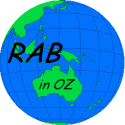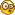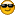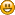# IN SEARCH OF PHEW THEOREM :D

• 47 Replies
• 1484 Views#### Danang

• 3005
• Everything will be "Phew" in its time :')##### IN SEARCH OF PHEW THEOREM :D
« on: May 06, 2019, 06:53:35 PM »
#EDITTED

===============================

For radius = 1, let's play the game of 1/2 Phew AKA 90° arc segment AKA 1.58578. If the circle line has the ratio of 0.79289 of the diameter, so the value of that arc length equals  1.58578 : √0.79289 AKA 1.78089.
Proof: 1.78089 × 1.78089 equals 3.17157 which is the circle area.

Let's calculate (especially)  the SPHERE AREA. (The sphere volume calculation will be straight 1.58578 as it is).

The former formula >> sA= (3.17157×6.34314)× 0.79289^2 = 12.6476 is not too accurate because there's two type of realities in spherical area to deal with, i.e.
1. Two poles (domes) with 1.58578 of diameters.
2. One curved Tape around equator with wide of 1.58578.

Let me introduce the concept of 0.890445517 which equals √0.79289

Curved Tape = wide × 0.890445517 x C = 1.58578 × 0.890445517 × 6.34314 = 8.9568.

The Polar Dome area = 1.58578^2 × 0.890445517^2 × √0.890445517 = 1.881515

Total Sphere Area = Curved Tape + 2 Polar Dome areas = 8.9568 + 2 [1.881515] = 12.71991

A little bit more than 4 Phew >> 4.01 times of Phew.

This is the clearest explanation ever.
Consider this is just a ~Cuick Count~. See you next in the ~Real Count~.

VIVA JOKOWI !!!===============================

This post....  👆👆👆👆👆 is just an EXPERIMENT, and I returned to the OLD THEOREM:

👇👇👇👇👇

https://www.theflatearthsociety.org/forum/index.php?topic=81376.msg2174834#msg2174834
« Last Edit: June 05, 2019, 04:00:25 PM by Danang »
TRY: Phew = 3.17157 and (Curved Grided) South Pole Centered FE Map AKA Phew FE Map and Phew Downwards Universal DeAcceleration.

Phew's Silicon Valley : https://gwebanget.home.blog/#### Danang

• 3005
• Everything will be "Phew" in its time :')##### Re: PHEW THEOREM FOUND
« Reply #1 on: May 06, 2019, 07:06:33 PM »
Note for the pole:
Area = [ ( 1.58578 × 0.79289 ) : √0.79289 ]^2 = [1.2573 : √0.79289]^2 = 1.9939
TRY: Phew = 3.17157 and (Curved Grided) South Pole Centered FE Map AKA Phew FE Map and Phew Downwards Universal DeAcceleration.

Phew's Silicon Valley : https://gwebanget.home.blog/#### rabinoz

• 21640
• Real Earth Believer##### Re: PHEW THEOREM FOUND
« Reply #2 on: May 06, 2019, 07:29:40 PM »
For radius = 1, let's play the game of 1/2 Phew AKA 90° arc segment AKA 1.58578. If the circle line has the ratio of 0.79289 of the diameter, so the value of that arc length equals  1.58578 : √0.79289 AKA 1.78089.
Proof: 1.78089 × 1.78089 equals 3.17157 which is the circle area.

Let's calculate (especially)  the SPHERE AREA. (The sphere volume calculation will be straight 1.58578 as it is).

The former formula >> sA= (3.17157×6.34314)× 0.79289^2 = 12.6476 is not too accurate because there's two type of realities in spherical area to deal with, i.e.
1. Two poles with 1.58578 of diameters.
2. Curved Tape around equator with wide of 1.58578.

The poles area = 1.58578 × √0.79289 times 1.58578 × √0.79289 = 1.9939
Two poles = 2 × 1.9939 = 3.7978.

Curved Tape = wide × √0.79289 x C =
1.58578 × √0.79289 × 6.34314 = 12.966468.
A little bit more than 4 Phew >> 4.0877 times of Phew.

This is the clearest explanation ever.
Consider this is just a ~Cuick Count~. See you next in the ~Real Count~.

VIVA JOKOWI !!!OK, when you get your "PHEW THEOREM" published in The International Journal of Applied Mathematics and Computer Science let's know and we'll look at it.

In the meantime, bye bye.##### Re: PHEW THEOREM FOUND
« Reply #3 on: May 06, 2019, 11:56:46 PM »
So to summarize: "If the ratio of the circumference of a circle to its diameter is phew (3.17156), then that PROVES the ratio of the circumference of a circle to its diameter is phew (3.17156).

Cool.#### Danang

• 3005
• Everything will be "Phew" in its time :')##### Re: PHEW THEOREM FOUND
« Reply #4 on: May 07, 2019, 02:38:45 AM »
For radius = 1, let's play the game of 1/2 Phew AKA 90° arc segment AKA 1.58578. If the circle line has the ratio of 0.79289 of the diameter, so the value of that arc length equals  1.58578 : √0.79289 AKA 1.78089.
Proof: 1.78089 × 1.78089 equals 3.17157 which is the circle area.

Let's calculate (especially)  the SPHERE AREA. (The sphere volume calculation will be straight 1.58578 as it is).

The former formula >> sA= (3.17157×6.34314)× 0.79289^2 = 12.6476 is not too accurate because there's two type of realities in spherical area to deal with, i.e.
1. Two poles with 1.58578 of diameters.
2. Curved Tape around equator with wide of 1.58578.

The poles area = 1.58578 × √0.79289 times 1.58578 × √0.79289 = 1.9939
Two poles = 2 × 1.9939 = 3.7978.

Curved Tape = wide × √0.79289 x C =
1.58578 × √0.79289 × 6.34314 = 12.966468.
A little bit more than 4 Phew >> 4.0877 times of Phew.

This is the clearest explanation ever.
Consider this is just a ~Cuick Count~. See you next in the ~Real Count~.

VIVA JOKOWI !!!OK, when you get your "PHEW THEOREM" published in The International Journal of Applied Mathematics and Computer Science let's know and we'll look at it.

In the meantime, bye bye.

Thank you Rabinoz... That's a good advise.
TRY: Phew = 3.17157 and (Curved Grided) South Pole Centered FE Map AKA Phew FE Map and Phew Downwards Universal DeAcceleration.

Phew's Silicon Valley : https://gwebanget.home.blog/#### Danang

• 3005
• Everything will be "Phew" in its time :')##### Re: PHEW THEOREM FOUND
« Reply #5 on: May 07, 2019, 02:44:09 AM »
So to summarize: "If the ratio of the circumference of a circle to its diameter is phew (3.17156), then that PROVES the ratio of the circumference of a circle to its diameter is phew (3.17156).

Cool.

Thank you Curiouser and Curiouser... This is a nice support. Sometimes I need the other's confirmation to convince myself.
TRY: Phew = 3.17157 and (Curved Grided) South Pole Centered FE Map AKA Phew FE Map and Phew Downwards Universal DeAcceleration.

Phew's Silicon Valley : https://gwebanget.home.blog/##### Re: PHEW THEOREM FOUND
« Reply #6 on: May 07, 2019, 11:52:04 AM »
So to summarize: "If the ratio of the circumference of a circle to its diameter is phew (3.17156), then that PROVES the ratio of the circumference of a circle to its diameter is phew (3.17156).

Cool.

Thank you Curiouser and Curiouser... This is a nice support. Sometimes I need the other's confirmation to convince myself.

Your understanding of propositional logic is as flawless as your mathematics.#### Danang

• 3005
• Everything will be "Phew" in its time :')##### Re: PHEW THEOREM FOUND
« Reply #7 on: May 08, 2019, 03:02:57 AM »
So to summarize: "If the ratio of the circumference of a circle to its diameter is phew (3.17156), then that PROVES the ratio of the circumference of a circle to its diameter is phew (3.17156).

Cool.

Thank you Curiouser and Curiouser... This is a nice support. Sometimes I need the other's confirmation to convince myself.

Your understanding of propositional logic is as flawless as your mathematics.

Thanks again. This even made me more confident with Phew. Although it's still needed more effort to figure out the exact value of sA.
« Last Edit: May 26, 2019, 02:29:21 PM by Danang »
TRY: Phew = 3.17157 and (Curved Grided) South Pole Centered FE Map AKA Phew FE Map and Phew Downwards Universal DeAcceleration.

Phew's Silicon Valley : https://gwebanget.home.blog/#### Danang

• 3005
• Everything will be "Phew" in its time :')##### Re: PHEW THEOREM FOUND
« Reply #8 on: May 08, 2019, 03:05:24 AM »
OK, when you get your "PHEW THEOREM" published in The International Journal of Applied Mathematics and Computer Science let's know and we'll look at it.

In the meantime, bye bye.

Please tell me how to do it.
TRY: Phew = 3.17157 and (Curved Grided) South Pole Centered FE Map AKA Phew FE Map and Phew Downwards Universal DeAcceleration.

Phew's Silicon Valley : https://gwebanget.home.blog/

?

#### Themightykabool

• 2150##### Re: PHEW THEOREM FOUND
« Reply #9 on: May 09, 2019, 02:40:05 PM »
Why wont you use a number that doesnt equal itself when squared cubed etc#### Danang

• 3005
• Everything will be "Phew" in its time :')##### Re: PHEW THEOREM FOUND
« Reply #10 on: May 10, 2019, 03:36:26 AM »
Why wont you use a number that doesnt equal itself when squared cubed etc

What number did you mean?
TRY: Phew = 3.17157 and (Curved Grided) South Pole Centered FE Map AKA Phew FE Map and Phew Downwards Universal DeAcceleration.

Phew's Silicon Valley : https://gwebanget.home.blog/

?

#### Themightykabool

• 2150##### Re: PHEW THEOREM FOUND
« Reply #11 on: May 10, 2019, 06:06:18 AM »
Why wont you use a number that doesnt equal itself when squared cubed etc

What number did you mean?

Ive told you a bunch of times.
R = 1.
Use something like 5 or 7 or 4.678.#### Danang

• 3005
• Everything will be "Phew" in its time :')##### Re: PHEW THEOREM FOUND
« Reply #12 on: May 10, 2019, 04:11:49 PM »
Why wont you use a number that doesnt equal itself when squared cubed etc

What number did you mean?

Ive told you a bunch of times.
R = 1.
Use something like 5 or 7 or 4.678.

r=1 is the standard for a constant.

Spherical area constant equals 12.94468.
If the radius = x so the spherical area for such radius equals x^2 times 12.94468

For instance r=5
sA = 5^2 × 12.94468 = 323.617
TRY: Phew = 3.17157 and (Curved Grided) South Pole Centered FE Map AKA Phew FE Map and Phew Downwards Universal DeAcceleration.

Phew's Silicon Valley : https://gwebanget.home.blog/

?

#### Themightykabool

• 2150##### Re: PHEW THEOREM FOUND
« Reply #13 on: May 10, 2019, 04:34:25 PM »
Yes.
But when you compare your measured values against your math you will see a bigger variance.
15×2×pi vs 15×2×phew.
That will, as many pointed out in your failed cone and circum posts, that pi falls within reasonable measuring error.
You got some wackoness thinking youre reinventing math.
Seriously.

« Last Edit: May 10, 2019, 04:36:23 PM by Themightykabool »#### Danang

• 3005
• Everything will be "Phew" in its time :')##### Re: PHEW THEOREM FOUND
« Reply #14 on: May 10, 2019, 11:53:36 PM »
Let's stick to reality.
The volume of a sphere equals +/- 50% of cube volume. For r=1, the magnitude is 8×50% = 4.

This number will ruin every pi based maths & formulas.
Because:

It approximately equals 3 pyramids with volume of 1.3334 each and 4 cones with volume of 1 each. (both h =1)

The volume ratio for cone : pyramid = 3:4 or 0.75 : 1.

In pi calculation this ratio doesn't match >> cone : pyramid = 1.047197 : 1.333334 = 0.785397 : 1.

Phew calculation:
Cone : Pyramid = 0.99695 : 1.33334 = 0.7477 : 1.
Close to 0.75 : 1. Yes because the exact volume of a sphere by experiment is slightly somewhere under 50% of a cube or under 4.
Phew claims it's 3.9878.

TRY: Phew = 3.17157 and (Curved Grided) South Pole Centered FE Map AKA Phew FE Map and Phew Downwards Universal DeAcceleration.

Phew's Silicon Valley : https://gwebanget.home.blog/#### Danang

• 3005
• Everything will be "Phew" in its time :')##### Re: PHEW THEOREM FOUND
« Reply #15 on: May 15, 2019, 01:50:32 AM »
Well, the dome areas on both poles is not 1.9939 actually.
The round object cannot be identical with the flat circle object if both have the same diameter.
The exact area of the dome equals 1.9939 times √0.890445517 AKA √(√0.79289) which results (by more precision in decimals) >> 1.881515

So the final result of Sphere Area equals 12.71991.
« Last Edit: May 15, 2019, 01:52:12 AM by Danang »
TRY: Phew = 3.17157 and (Curved Grided) South Pole Centered FE Map AKA Phew FE Map and Phew Downwards Universal DeAcceleration.

Phew's Silicon Valley : https://gwebanget.home.blog/

?

#### Themightykabool

• 2150##### Re: PHEW THEOREM FOUND
« Reply #16 on: May 15, 2019, 03:13:45 AM »
Let's stick to reality.
The volume of a sphere equals +/- 50% of cube volume. For r=1, the magnitude is 8×50% = 4.

This number will ruin every pi based maths & formulas.
Because:

It approximately equals 3 pyramids with volume of 1.3334 each and 4 cones with volume of 1 each. (both h =1)

The volume ratio for cone : pyramid = 3:4 or 0.75 : 1.

In pi calculation this ratio doesn't match >> cone : pyramid = 1.047197 : 1.333334 = 0.785397 : 1.

Phew calculation:
Cone : Pyramid = 0.99695 : 1.33334 = 0.7477 : 1.
Close to 0.75 : 1. Yes because the exact volume of a sphere by experiment is slightly somewhere under 50% of a cube or under 4.
Phew claims it's 3.9878.

3.99 is also under 4.
this is beyond stupid.#### Danang

• 3005
• Everything will be "Phew" in its time :')##### Re: PHEW THEOREM FOUND
« Reply #17 on: May 15, 2019, 08:05:36 AM »
Oops...

TRY: Phew = 3.17157 and (Curved Grided) South Pole Centered FE Map AKA Phew FE Map and Phew Downwards Universal DeAcceleration.

Phew's Silicon Valley : https://gwebanget.home.blog/#### sokarul

• 15840
• Discount Chemist##### Re: PHEW THEOREM FOUND
« Reply #18 on: May 15, 2019, 05:15:28 PM »
Well, the dome areas on both poles is not 1.9939 actually.
The round object cannot be identical with the flat circle object if both have the same diameter.
....
You used to preach they were the same. Finally you figured out you are wrong.
Sokarul

ANNIHILATOR OF  SHIFTER#### Danang

• 3005
• Everything will be "Phew" in its time :')##### Re: PHEW THEOREM FOUND
« Reply #19 on: May 15, 2019, 05:52:51 PM »
Well, the dome areas on both poles is not 1.9939 actually.
The round object cannot be identical with the flat circle object if both have the same diameter.
....
You used to preach they were the same. Finally you figured out you are wrong.

It's normal.
The thing is, we move forwards till the final conclusion obtained.
At least the logic of "curved tape" and "dome" is something fresh and can become the basis of further calculation.
At least Phew Theorem covers such sphere reality.
TRY: Phew = 3.17157 and (Curved Grided) South Pole Centered FE Map AKA Phew FE Map and Phew Downwards Universal DeAcceleration.

Phew's Silicon Valley : https://gwebanget.home.blog/#### Danang

• 3005
• Everything will be "Phew" in its time :')##### Re: PHEW THEOREM FOUND
« Reply #20 on: May 16, 2019, 12:33:56 AM »
To go to details:

Within the curved tape, there are actually four fractions of squared curved tape with length of 1.58578 each.
Let's call this the curved square.

Curved square area = 0.890445517 × 1.58578^2 = 2.23922

And then to define the dome's area, we'll find first the circle flat area within the square of 1.58578^2 >> time it with 0.79289 or 0.890445517^2. The result will be 1.9939.

Since this circle area is within the square of 1.58578^2 whose rounding scale equals 0.890445517, so the circle area's rounding scale will be √0.890445517.
Thus the polar dome area equals 1.9939 times √0.890445517 = 1.881515.

Conclusion:
1. To form a curved square from a square of 1.58578^2, it takes the multiplier >> 0.890445517.
2. To form a polar dome from a circle area within the square 1.58578^2, it takes the multiplier >> √0.890445517.
« Last Edit: May 16, 2019, 03:02:41 PM by Danang »
TRY: Phew = 3.17157 and (Curved Grided) South Pole Centered FE Map AKA Phew FE Map and Phew Downwards Universal DeAcceleration.

Phew's Silicon Valley : https://gwebanget.home.blog/#### Danang

• 3005
• Everything will be "Phew" in its time :')##### Re: PHEW THEOREM FOUND
« Reply #21 on: May 16, 2019, 12:47:42 AM »
Let's compare areas within the square (√2)^2:

(A) The flat circle area.
(B) The cone area.
(C) The polar dome area.
Plus
(D) The flat circle area within 1.58578^2.

A : B : C : D = 1.58578 : 1.71644: 1.881515 : 1.9939.

« Last Edit: May 16, 2019, 12:49:55 AM by Danang »
TRY: Phew = 3.17157 and (Curved Grided) South Pole Centered FE Map AKA Phew FE Map and Phew Downwards Universal DeAcceleration.

Phew's Silicon Valley : https://gwebanget.home.blog/#### Danang

• 3005
• Everything will be "Phew" in its time :')##### Re: IN SEARCH OF PHEW THEOREM :D
« Reply #22 on: May 29, 2019, 02:14:38 AM »
FINALLYSometimes you quit something and then return.
I also quit Phew for a while coz I thought it's wrong, in fact it's awesome.

The same case with sA = 2 [ Phew^2 × 0.79289^2 ] = 12.6476.

It's clearer and logical.

It can be explained by the hint numbers within perimeter, among others: "1.58578" or 1/2 Phew AKA 90° of Circumference.
It's 2 × 1.58578^4 = 12.647619 (by detailed decimals).

Or hemisphere area times 2 >> [ C × 0.79289 times curved height × 0.79289 ] times 2.

The idea:
C grows smaller into zero through the path length of 1.58578 by the scale 0.79289. Either C and the path of 1.58579 got cut by 0.79289 each. It applies for a hemisphere. Total: time it with 2.

CASE CLOSEDTRY: Phew = 3.17157 and (Curved Grided) South Pole Centered FE Map AKA Phew FE Map and Phew Downwards Universal DeAcceleration.

Phew's Silicon Valley : https://gwebanget.home.blog/#### Bullwinkle

• Flat Earth Curator
• 16257
• "Umm, WTF ???"##### Re: IN SEARCH OF PHEW THEOREM :D
« Reply #23 on: May 29, 2019, 10:19:33 PM »
Congratulations.RE can never win this argument.
FE can't be disproved.#### Danang

• 3005
• Everything will be "Phew" in its time :')##### Re: IN SEARCH OF PHEW THEOREM :D
« Reply #24 on: May 30, 2019, 04:19:57 AM »
Congratulations.Thaaank youuuu dude..!!

The next step is... PHEW APP.

Are you, or anyone familiar with programming?
TRY: Phew = 3.17157 and (Curved Grided) South Pole Centered FE Map AKA Phew FE Map and Phew Downwards Universal DeAcceleration.

Phew's Silicon Valley : https://gwebanget.home.blog/#### Bullwinkle

• Flat Earth Curator
• 16257
• "Umm, WTF ???"##### Re: IN SEARCH OF PHEW THEOREM :D
« Reply #25 on: May 30, 2019, 04:24:13 AM »
Congratulations.Thaaank youuuu dude..!!

The next step is... PHEW APP.

Are you, or anyone familiar with programming?

I am "familiar" with programming.RE can never win this argument.
FE can't be disproved.#### NotSoSkeptical

• 4895
• The Caretaker##### Re: IN SEARCH OF PHEW THEOREM :D
« Reply #26 on: May 30, 2019, 04:42:28 PM »
If you are searching for the phew theorem, then how did you come up with your number.
All work and no play makes Jack a dull boy#### Danang

• 3005
• Everything will be "Phew" in its time :')##### Re: IN SEARCH OF PHEW THEOREM :D
« Reply #27 on: May 30, 2019, 06:27:41 PM »
Congratulations.Thaaank youuuu dude..!!

The next step is... PHEW APP.

Are you, or anyone familiar with programming?

I am "familiar" with programming.Cool..! What are you gonna do?

The requirements for those who wish to create the Phew app are simple:
1. Write to citizenofatlantis[at]gmail.com for lisence request.
2. The statement that you will mention "Phew" in the title of the app as well as my name as the Phew founder in the app info, besides your name as the author of the application.
3. The profit shares statement... How much?? Okay, let your own costume decide what the shares is like.

If you or anybody else are agree, please email me.
« Last Edit: May 30, 2019, 08:36:44 PM by Danang »
TRY: Phew = 3.17157 and (Curved Grided) South Pole Centered FE Map AKA Phew FE Map and Phew Downwards Universal DeAcceleration.

Phew's Silicon Valley : https://gwebanget.home.blog/#### Danang

• 3005
• Everything will be "Phew" in its time :')##### Re: IN SEARCH OF PHEW THEOREM :D
« Reply #28 on: May 30, 2019, 06:33:51 PM »
If you are searching for the phew theorem, then how did you come up with your number.

I found the formulas at least based on:
1. Logical calculations.
2. Hint figures within a perimeter under a definite square with all mindblowing 'coincidence'.
3. Real experiments.
TRY: Phew = 3.17157 and (Curved Grided) South Pole Centered FE Map AKA Phew FE Map and Phew Downwards Universal DeAcceleration.

Phew's Silicon Valley : https://gwebanget.home.blog/#### Danang

• 3005
• Everything will be "Phew" in its time :')##### Re: IN SEARCH OF PHEW THEOREM :D
« Reply #29 on: May 30, 2019, 06:49:03 PM »
Examples:
Because there have been tiny arc segments abandoned, Pi must be somewhere above 3.14159.
Phew? >> Ratio 45° Arc : Radius = 0.79289 : 1.
0.78289 comes from >> (Sin45) + (1-Cos45)^2 OR (Sin45)^2 + (1-Cos45)

With the same reason -by pyramid base area method- the spherical area MUST be LESS than 4 circle area AKA 4 Pi. (Too bad Pi is wrong).
Phew says it's 3.987806 times Phew AKA 12.647619.

Also 3.987806 is the exact number of spherical volume, instead of something significantly more than 50% (=4.188) of a cube volume. Experiment says so, even though extremely rare in Youtube. Usually they use cylinder as measurement tool, instead of cube.
« Last Edit: May 30, 2019, 06:51:07 PM by Danang »
TRY: Phew = 3.17157 and (Curved Grided) South Pole Centered FE Map AKA Phew FE Map and Phew Downwards Universal DeAcceleration.

Phew's Silicon Valley : https://gwebanget.home.blog/